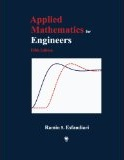## Atlantis Publishing Company (APC)Academic and Reference Textbooks

As a publisher of academic and reference textbooks, APC is committed to providing innovative and effective instructional materials for higher education.

#Applied Mathematics for Engineers, Fifth Edition

## Book Description

• Price: U.S. \$139.99
• ISBN: 978-0-9729990-7-6
• 662 pp., hard cover, June 2013
• Inside this Book
Order

The principal goal of this book is to provide the reader with a comprehensive knowledge of fundamental concepts and methods of applied mathematics used in different engineering disciplines. Each topic is covered in great detail, followed by several meticulously worked-out examples, as well as a problem set containing a large number of additional related exercises. Engineering applications are stressed and integrated throughout the book, and consistent with that notion, derivations of the mathematical models of many physical systems are included to familiarize the reader with the basic laws and proper engineering terminology. The fourth edition of the book (2007) has been entirely revamped to produce this latest edition through the addition of several topics and removal of a few others.

The book is essentially divided into three main parts. The first part covers complex analysis (Chapter 1), ordinary and partial differential equations (Chapters 2-4 and 7), Laplace transformation (Chapter 5), and Fourier analysis (Chapter 6). In the second part, a detailed coverage of matrix analysis (Chapter 8) and matrix eigenvalue problem (Chapter 9) is presented. Several applications in engineering vibrations are included for a better understanding of the effectiveness of matrix computations in determining systems natural frequencies and mode shapes. The third part of the book is completely devoted to numerical methods with applications in different engineering disciplines.

The powerful software MATLAB� is introduced in Chapter 10 and is used to perform symbolic, graphical, and numerical tasks. Chapter 11 introduces techniques for solving equations and systems. Chapter 12 discusses curve fitting and interpolation techniques, while Chapter 13 covers numerical differentiation and integration methods. Chapter 14 presents numerical methods for solving initial- and boundary-value problems. Chapter 15 introduces methods to approximate eigenvalues of a matrix. Numerical solution of partial differential equations is covered in Chapter 16.